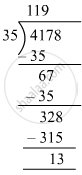# Divide, and find out the quotient and remainder. Check your answer. 4178 ​÷ 35 - Mathematics

Fill in the Blanks

4178 ​÷ 35

#### SolutionDividend = 119 and Remainder = 13
Check: Divisor × Quotient + remainder = 35 × 119 + 13
= 4178
= Dividend
∴ Dividend= Divisor × Quotient + Remainder

Is there an error in this question or solution?

#### APPEARS IN

RS Aggarwal Class 6 Mathematics
Chapter 3 Whole Numbers
Exercise 3E | Q 2.2 | Page 56